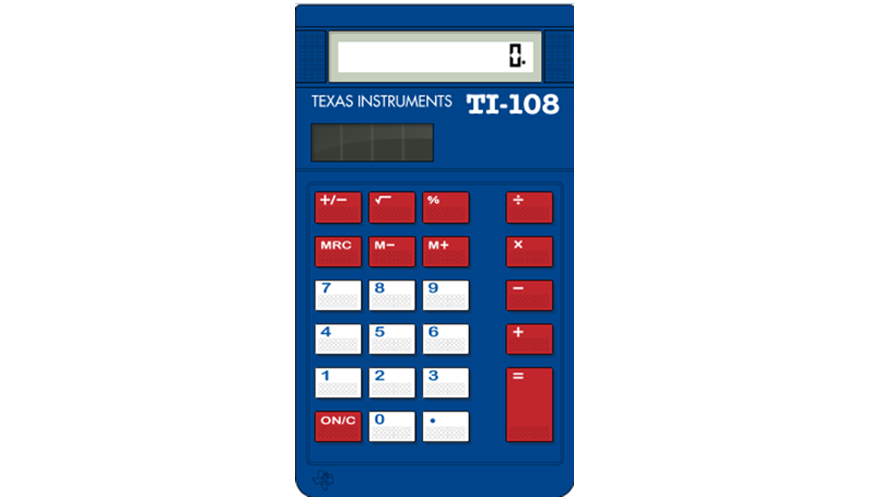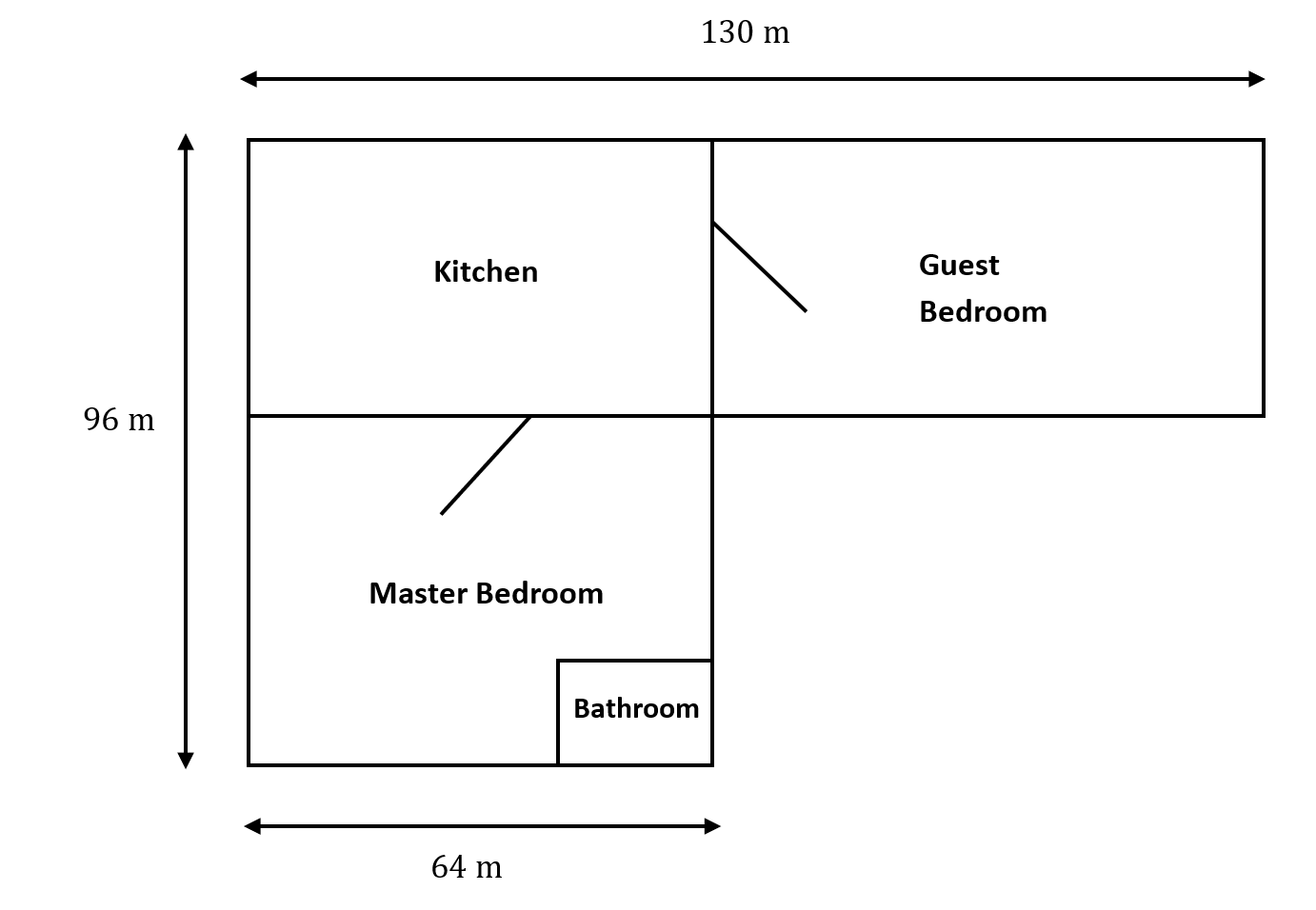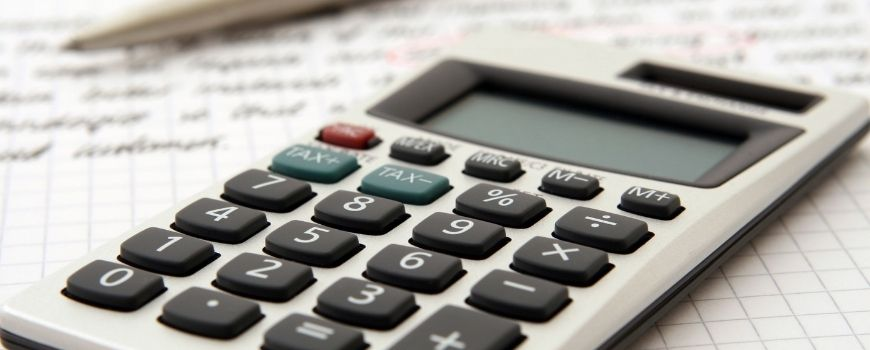# 5 Things You Must Know About the UCAT Calculator

To ace the UCAT, you need to be familiar with the on-screen calculator. Read this article to learn 5 things you must know about the UCAT calculator.

While you are not allowed to bring in your own calculator or any other electronic devices, the UCAT provides a basic on-screen calculator for the decision making and quantitative reasoning subtests. You can access this calculator by clicking the icon at the top left of the screen.

## 5 things you must know about the UCAT calculator

There are some key things about the calculator that trip up students sitting the UCAT. Here are the 5 most important things you should know about the UCAT calculator.## 1. The UCAT calculator does not consider the order of operations

You might remember the order of operations as BODMAS (Brackets Order Division Multiplication Addition Subtraction), but the calculator disregards this convention. It instead applies the operations in whatever order you enter it as. This potentially results in a significantly different answer, which, if unaccounted for, could make all your calculations incorrect.

For example, consider: $12 - 4 \times 2 = ?$

Since multiplication is prioritised before subtraction in the order of operations:

$12 - 4 \times 2$
$= 12 - 8$
$= 4$

However, the UCAT calculator will simply read the expression from left-to-right to evaluate it as:

$12 - 4 \times 2$
$= (12 - 4) \times 2$
$= 8 \times 2$
$= 16$

The diagram (not to scale) below outlines the floor plan for a collection of new apartments:• The bathroom makes up 25% of the combined area of both the kitchen and guest bedroom.
• The cost of covering the floors with carpeting is priced at \$6 per square metre

If the perimeter of the master bedroom (including the bathroom) is $390m^2$, what is the width of that particular bedroom?

Solution:
Let y be the length of the left side of the master bedroom.

Then, we have

$2 × y + 2 × 64 = 268$
$2y = 268 - 2 × 64$
$y = \frac{(268 - 2 × 64)}{2}$

By considering the order of operations, we would reach our correct answer of:

$y$
$= \frac{(268 - 128)}{2}$
$= \frac{140}{2}$
$= 70$

However, if we were to just enter the expression into the UCAT calculator, we would reach the incorrect answer of:

$y$
$= \frac{((268 - 2) × 64)}{2}$
$= \frac{(266 × 64)}{2}$
$= 8512$

As you can see, you must be careful with your calculations and ensure that you are not relying on the calculator to manage your order of operations. Otherwise, you could end up with a bizarre result, which you hopefully will realise is very much incorrect.## 2. The calculator can store values.

If you think you may need to repeatedly use a value that is not easy to remember or enter, it could be worth storing it into the calculator’s memory.

The M+ button saves the number present on the calculator display to memory, while the M- button saves the negative value of the number. This value can be recalled from the memory by clicking the MRC button. The memory will be cleared if you press MRC with M-.

Note that the calculator can only store one value at a time, which means that saving another number will overwrite the previous number saved. So, make sure to jot down any numbers that you think you may need for later calculations.## 3. The display screen will clear when you move to a different question

This is probably a convenient function as most questions will not require values from previous questions. However, when there are multiple questions linked together, you will need to remind yourself to write down any relevant values on your laminated whiteboard to avoid redoing the calculation for later questions. Time is definitely limited in the UCAT, so you won’t want to waste any of it repeating yourself.

## 4. You can use the number pad on your keyboard to input numbers and operations

Using the mouse to click the buttons on the on-screen calculator can be quite fiddly and time-consuming, so we recommend that you utilise the number pad on the right-hand side of your keyboard. While most testing centres will have keyboards with number pads, some won’t, so it’s best to also practise using the numbers and operations at the top of your keyboard.

Please note that the ‘Num Lock’ must be on for the number pad to work. Not only can you enter numbers via the number pad, but you can also use specific keys for mathematical operations.

### Key Operation

forward slash ( / ) : division (÷)
asterisk ( * ) : multiplication ( × )
plus ( + ) : addition
minus ( – ) : subtraction

## 5. The backspace key clears the display screen.

The backspace key does not delete one number at a time, but rather, it has the same functionality as the ON/C button. This means that you should try to be accurate when you type, as all previous entries will all need to be cleared if you make a mistake. However, it’s completely fine if you do happen to mistype a value — just stay calm and be aware that you will need to type the entirety of the number again.

## Want to practise using the same calculator as in the actual exam?

The UCAT Masterclass exam simulator is the same as that used in the Pearson VUE testing centres. When you study UCAT with UCAT Masterclass you get to practise using the calculator, too. Sign up for a free trial account!